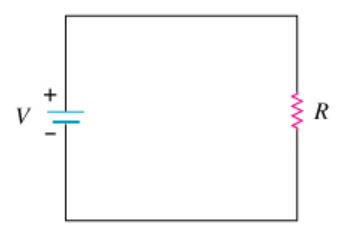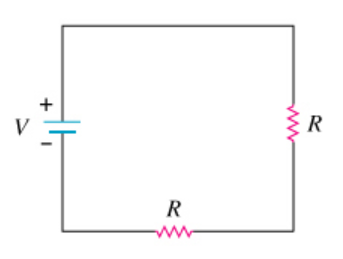# Problem: A single resistor is wired to a battery as shown in the diagram below.(Figure 1) Define the total power dissipated by this circuit as P0.Now, a second identical resistor is wired in series with the first resistor as shown in the second diagram to the leftWhat is the power, in terms of P0, dissipated by this circuit? Express your answer in terms of P0.

###### FREE Expert Solution

Power:

$\overline{){{\mathbf{P}}}_{{\mathbf{0}}}{\mathbf{=}}\frac{{\mathbf{V}}^{\mathbf{2}}}{\mathbf{R}}}$

In series, equivalent resistor:

Req = R1 + R2 = R + R = 2R

88% (349 ratings)###### Problem Details

A single resistor is wired to a battery as shown in the diagram below.(Figure 1) Define the total power dissipated by this circuit as P0.Now, a second identical resistor is wired in series with the first resistor as shown in the second diagram to the leftWhat is the power, in terms of P0, dissipated by this circuit? Express your answer in terms of P0.